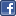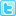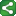Microsoft Excel Resource Center Free Macros, Formulas, Functions, Tutorials, Downloads, Add-Ins & More!
 Monte Carlo Tutorial power4XL Home
 Contents

Multi Coin Toss example VBA codeShareThis

Below is the code behind the VBA version of the Multi Coin Toss simulation.

Note that we do not retain the independent results of each iteration of the model.  This would consume large system resources for high numbers of iterations.  Instead, in each iteration we are simply summarizing and storing the cumulative results in the HeadCount(11) array.

The array has 11 elements, since there are 11 possible outcomes of tossing a coin ten times (zero to ten heads).The code uses "nested loops" - one "for" loop for the number of iterations, and another "for" loop nested within the first one to toss the coin 10 times.